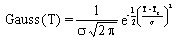# Volumetric heat capacity, exponential function of T + Gaussian of T

## Presentation

This model defines a volumetric heat capacity ρCp as an exponential function of the temperature, taking the energy of phase transition (Curie point, ...) into consideration.

## Mathematical model

The volumetric heat capacity is the combination of an exponential function and a Gaussian function of the temperature.

The corresponding mathematical formula is written:

withwhere:

• ρCp0 is a constant the volumetric heat capacity at T = 0 °C (J/m3/°C)
• ρCpi is another constant the volumetric heat capacity at T = infinite (J/m3/°C)

• E is the energy of phase transition (J/m3)
• σ is the typical Gaussian standard deviation (°C)
• TC is the transition temperature (Curie temperature, …) (°C)
• τ is a temperature constant of the exponential function (positive or negative) (°C)

The shape of ρCp(T) curve is presented in the figure below.